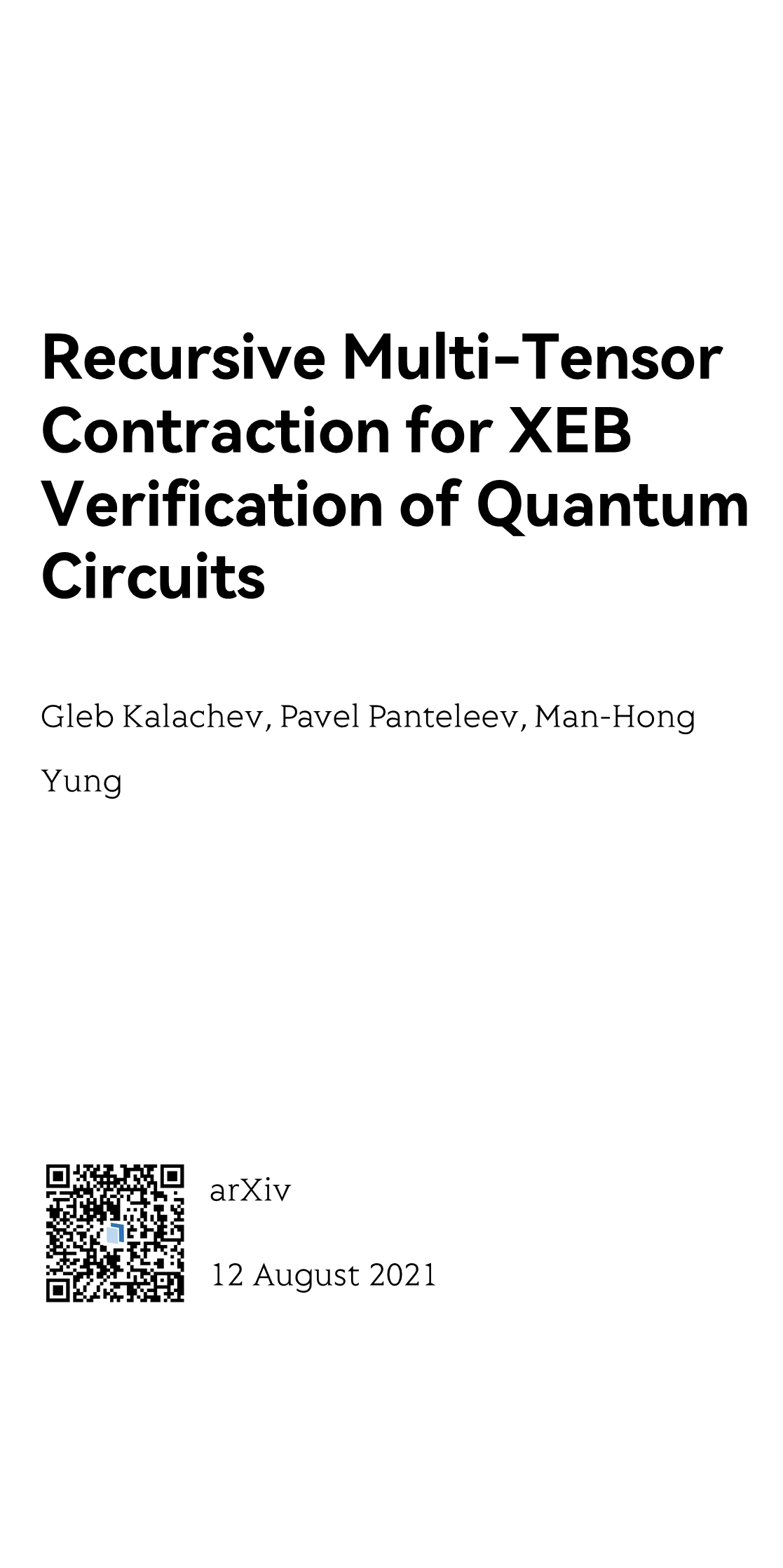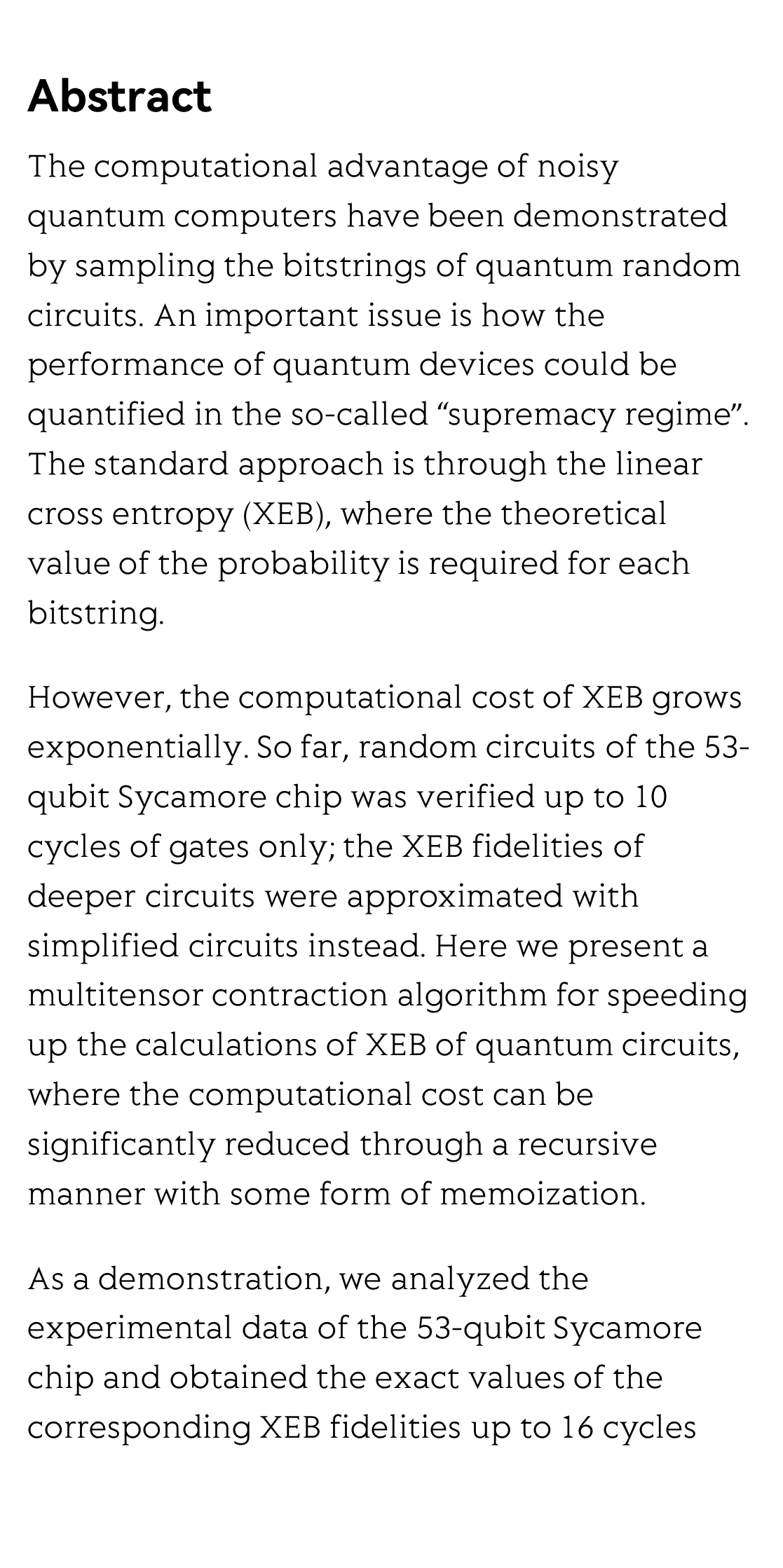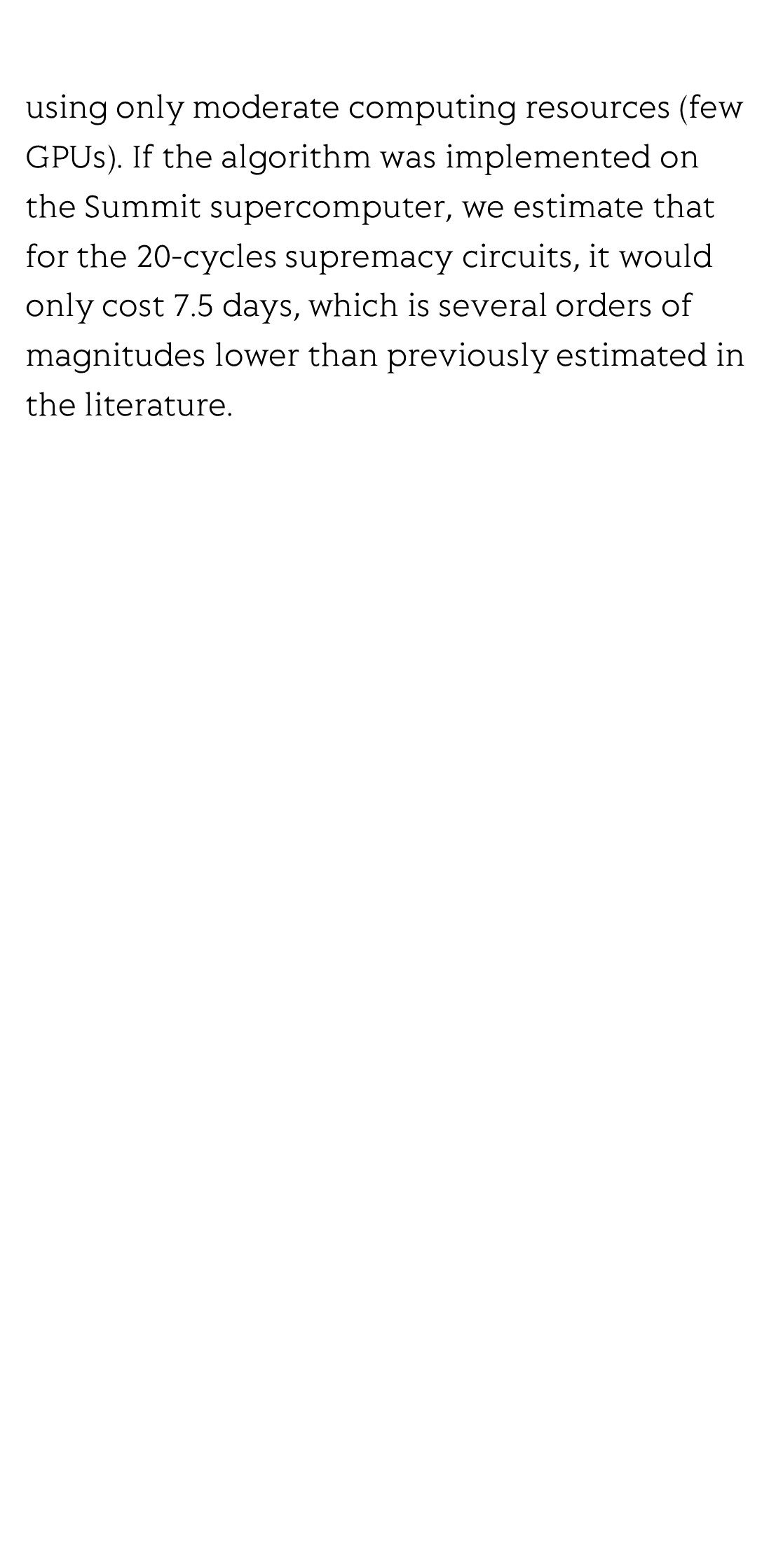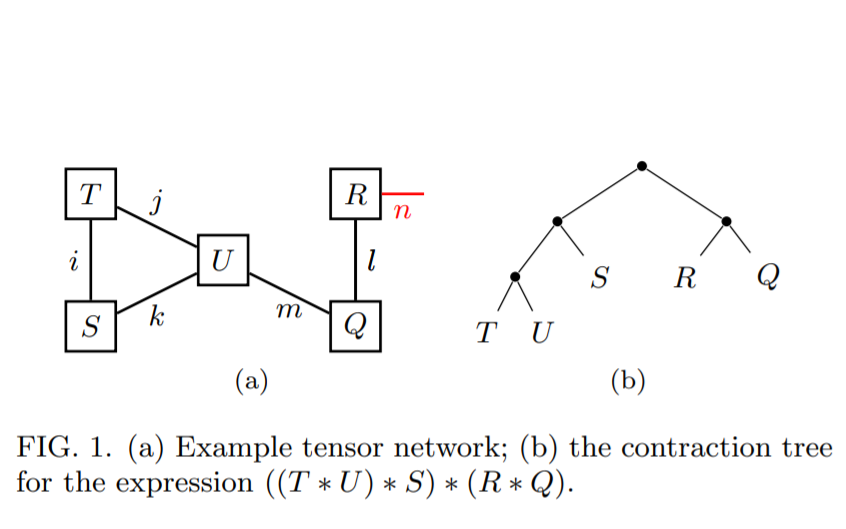MMMYYYYRecursive Multi-Tensor Contraction for XEB Verification of Quantum Circuits

양자 회로 XEB 검증 의 재 귀 다 장 량 압축
Compresión multitensor recursiva verificada por el circuito cuántico XEB
Compression multitensorielle récursive pour la vérification XEB des circuits quantiques
Рекурсивное мульти-тензорное сжатие для проверки квантовых схем с помощью XEB
Gleb Kalachev ¹ ², Pavel Panteleev ¹ ², Man-Hong Yung 翁文康 ¹ ³
¹ Huawei 2012 Lab

² Lomonosov Moscow State University
³ Institute for Quantum Science and Engineering, and Department of Physics, Southern University of Science and Technology, Shenzhen, 518055, China

arXiv, 12 August 2021Abstract

The computational advantage of noisy quantum computers have been demonstrated by sampling the bitstrings of quantum random circuits. An important issue is how the performance of quantum devices could be quantified in the so-called “supremacy regime”. The standard approach is through the linear cross entropy (XEB), where the theoretical value of the probability is required for each bitstring.

However, the computational cost of XEB grows exponentially. So far, random circuits of the 53-qubit Sycamore chip was verified up to 10 cycles of gates only; the XEB fidelities of deeper circuits were approximated with simplified circuits instead. Here we present a multitensor contraction algorithm for speeding up the calculations of XEB of quantum circuits, where the computational cost can be significantly reduced through a recursive manner with some form of memoization.

As a demonstration, we analyzed the experimental data of the 53-qubit Sycamore
chip and obtained the exact values of the corresponding XEB fidelities up to 16 cycles using only moderate computing resources (few GPUs). If the algorithm was implemented on the Summit supercomputer, we estimate that for the 20-cycles supremacy circuits, it would only cost 7.5 days, which is several orders of magnitudes lower than previously estimated in the literature.Reviews and Discussions